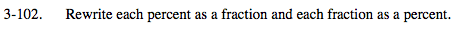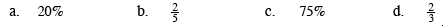### Home > MC2 > Chapter 3 > Lesson 3.2.4 > Problem3-102

3-102.A percent is a portion out of 100.
Given any percent, one can set it as a fraction over 100 and simplify to get the desired fraction.

$20\% \rightarrow \frac{20}{100}= \frac{1}{5}$

Now use the same method for part (c).

Given any fraction, divide the numerator by the denominator
to get the decimal form. At this point, multiply the decimal
by 100 to get the percent form.

$\frac{2}{5}=0.4 \rightarrow 40\%$

Now try to use the same method for part (d).